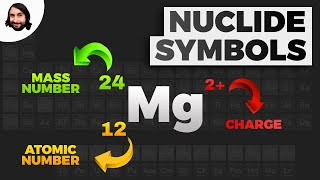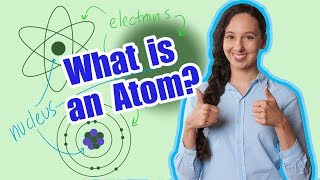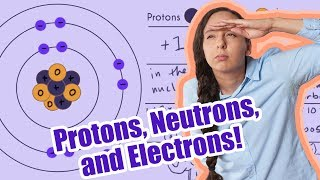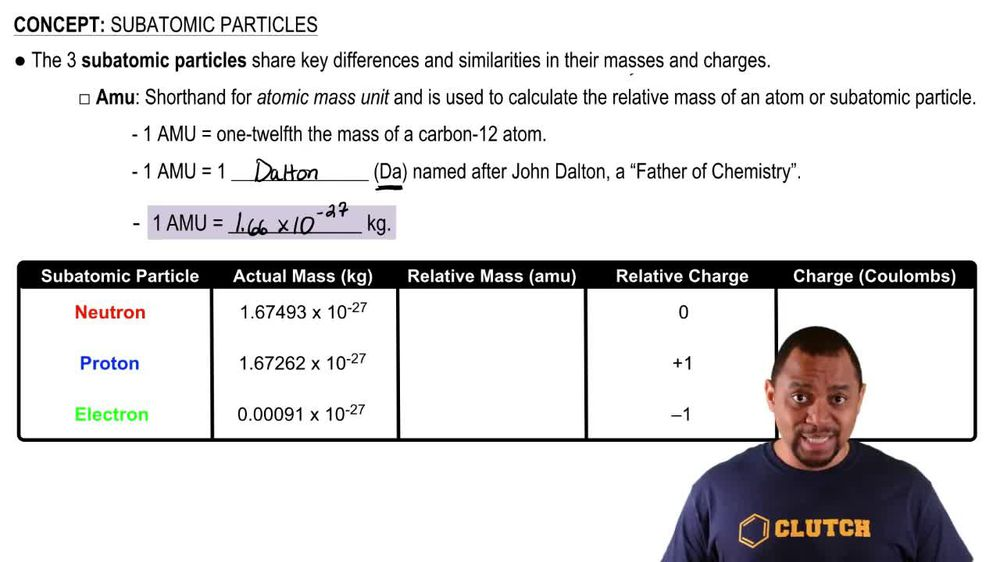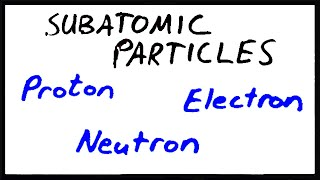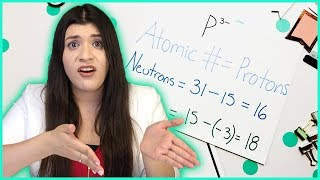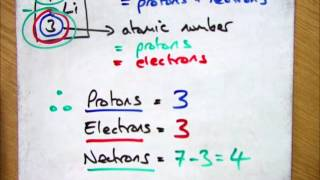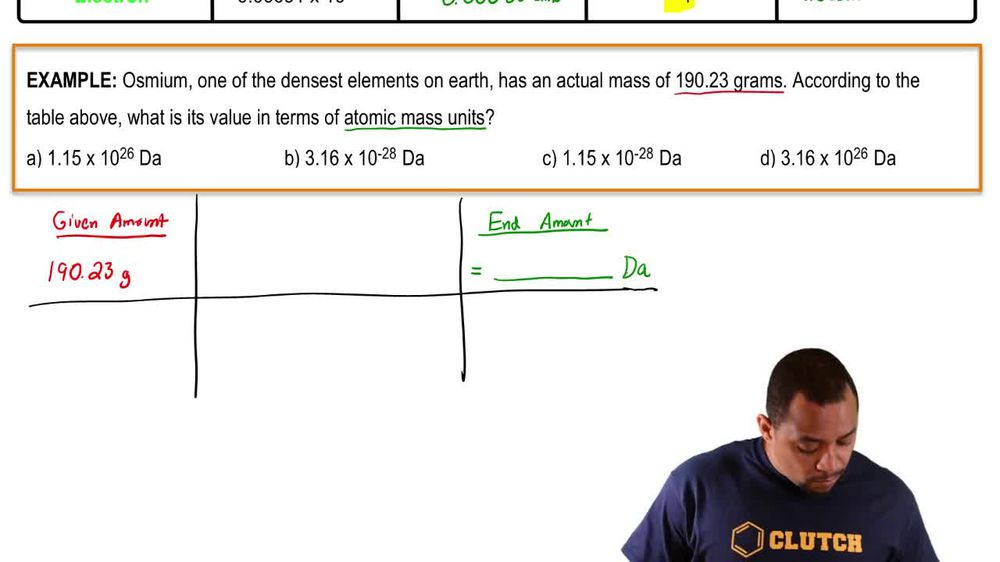Start typing, then use the up and down arrows to select an option from the list.
1. 2. Atoms & Elements2. Subatomic Particles
Problem

# Determine whether each of the following statements is true or false. If false, correct the statement to make it true: (c) The number of electrons in an atom equals the number of neutrons in the atom.

Relevant Solution1m
Play a video:
Hey everyone where us is a statement below, correct or incorrect. If it is false, modify the statement into a correct one. Our statement here says the atomic number of an element is determined by the number of neutrons. First. Let's go ahead and look at an example. We can write oxygen in its nuclear notation and as we can see right here, oxygen has eight protons, eight neutrons and eight electrons. Now, if we had an isotope of oxygen such as oxygen 17, We would have eight protons nine neutrons and eight electrons. So comparing these two, we know that this bottom number is our atomic number. So this statement is going to be false and the way to correct it is to remove the term neutrons and replace it with protons. So our final sentence is going to be, the atomic number of an element is determined by the number of protons. Now, I hope that made sense. And let us know if you have any questions.